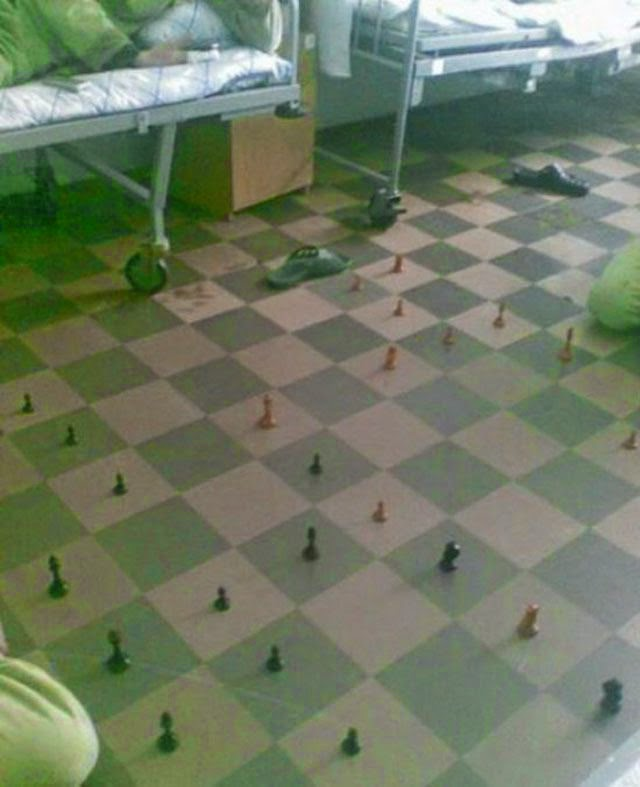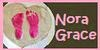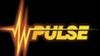## Posts

Showing posts from June, 2014

### Mars 1.91 - (strong) chess engines UCI (based on Ippolit) . New version 26.06.2014Mars - UCI chess engines

RatingsJCER older version:
Mars 1.5 - 3048, Mars 1.7 - 3041, Mars 1.1 - 2985, Mars 1 - 2911, Mars 1.8 - 2880, Mars 1.6 - 2830

### Jurek Chess Engines Rating. Test new chess engines: Deuterium 14.2.33.276, SmarThink 1.60, Rhetoric 1.4, Sjakk 2.1, NoraGrace 1.0 and Orion 0.2

2014.06.23-24 JCER Test New Versions Engines

REngineScoreDeSmRhGoSjChNoOrS-B1Deuterium 14.2.33.276 x6424.0/28· ·· ·==1=0=1111111=11=11111111111 276,50 2SmarThink 1.60 x64 24.0/28==0=· ·· ·==11111=1111111111111111 265,25 3Rhetoric 1.4 x64 18.5/281=00==00· ·· ·101=1011==1=11111111 188,25 4Godel 3.4.9 x64 16.5/280000000=010=· ·· ·=1=11=1111111111 137,75 5Sjakk 2.1 x64 12.5/280=0000000100=0=0· ·· ·01=1111111=1 95,25 6Cheese 1.61 x64 11.0/28=0000000==0=0=0010=0· ·· ·1=11111= 86,00 7NoraGrace 1.03.0/28000000000000000000000=00· ·· ·1=10 11,75 8Orion 0.22.5/28000000000000000000=0000=0=01· ·· · 16,25

112 games download, Temp: 3' + 3", Hardware: Intel Core i5-3210M CPU @ 2.50GHz with 7,9 GB Memory
Operating system: Windows 8.1 Home Edition (Build 9200) 64 bit book: Perfect 2014,  Table and GUI: Arena 3.5

### Hospital chess### SmarThink 1.60 - UCi chess engine. New version 23.06.2014SmarThink, - UCI chess engine
Author - Sergei S. Markoff,  website

### Sjakk 2.1 UCI chess engine. New version 23.06.2013Sjakk - UCI chess engine
Author - Jacob Børs Lind

JCER ratings older version: Sjakk 2.0 = 2305,

### Deuterium book - new version 21.06.2014### Deuterium 14.2.33.276 - UCI chess engine. New version 15.02.2014Deuterium - UCI chess engineWebsite
RatingsJCER older version:
Deuterium 13.1.31.113 = 2644
Deuterium 14.1.32.119 = 2629

### Houdini 4 wins test tournament Jurek Chess Engines Rating. 21-22.06.2014

JCER, TestTournament, 2014.06.21 - 2014.06.22

PEnginesScore Houd Gull 3StockCritt KomoStrel +/-/=1 Houdini 4 x64 23.5 / 40 XXXXX==1==11=000=========1=11==0=11===10111==+12 -5 =232 Gull 3 x64 22.5 / 40 ==0==00=XXXXX11==1=101001====0101=111=1==1===+13 -8 =193 Stockfish 160614 64 SSE4.2 22.0 / 40 111=====00==0=01XXXXX==1==1======1110===10===+10 -6 =244 Critter 1.6a 64-bit 19.0 / 40 ====0=000110======0==0==XXXXX1=00001111==1==1+9 -11 =205 Komodo 7a 64-bit 18.0 / 40 ==1=00==1010=000====00010=111100XXXX=10==1==+10 -14 =166 Strelka 6 w32 15.0 / 40 =01000===0==0======01===00==0==0=01==0==XXXXX+3 -13 =24120 games download, book: Perfect 2014,  Table and GUI:Fritz 12
Hardware: Intel(R) Core(TM) i5-3210M CPU @ 2.50GHz with 7,9 GB Memory
Operating system: Windows 8.1 Home Edition (Build 9200) 64 bit  TEMP 3' + 3"

### Komodo 7a wins Jurek Chess Engines Rating test tournament. 2014.06.21-22

JCER Test Tournament, 2014.06.21 - 2014.06.22

M
Engines Score (Tie) KomoFireGullStreCritDeepChirBlac 1: Komodo 7a 64-bit 18.5 / 28 231.00 XXX=010=0=0=11==1=11111=1011=11 2: Fire 3.0 x64 18.0 / 28 226.25 =101XXX=01===0===11==1=1111==1= 3: Gull 3 x64 17.5 / 28 218.25 =1=1=10=XXX011==00===101=1=1111 4: Strelka 6 w32 17.5 / 28 212.00 =00===1=100=XXX10==111111=11=1= 5: Critter 1.6a 64-bit 15.5 / 28 192.00 =0=0==00=11=01==XXX1=10===11=11 6: Deep Rybka 4.1 x64 13.0 / 28 133.25 0000==0===0100000=01XXX11111111 7: Chiron 2 64bit 6.5 / 28 88.25 =01000000=0=00=0===00000XXX=0=1 8: BlackMamba 2.0 x64 5.5 / 28 74.50 0=00==0=00000=0=0=000000=1=0XXX180 games download, Table and GUI:Fritz 14 Temp 3' + 3"
Hardware: Intel(R) Core(TM) i3-3217U CPU @ 1.80GHz z 3,9 GB RAM
Operating system: Windows 8.1 Home Edition (Build 9200) 64 bit

### Lucas Chess 8.08 new official version - best chess softwareYou have an extensive list of trainings with which to try to improve your chess:
- Training positions
- Play like a grandmaster
- Training mates
- Find best move
- Learn openings by repetition
- Training with a book
- Resistance Test
- Learn tactics by repetition
- Check your memory on a chessboard
- Find all moves
- Becoming a knight tamer
- Moves between two positions
- Learn a game

The program has 21 engines prepared to play from the start, and with very different levels, from 1300 to 3000 elo.
This list of engines is not closed and you can add other ones with the only limitation that they use the UCI protocol.
The game can be set, limiting the depth of analysis of the motor or the time used to think, or by modifying the way in which it decides. You can also choose the opening, or start in a certain position, or that the engine uses a book of openings or more or less aid.
Younger children will be able to begin their apprenticeship with sp…

### NoraGrace 1.0 - winboard chess engine. New version 21.06.2014NoraGrace 1.0 - winboard chess engine
Author - Eric Oldre

### Rhetoric 1.4 - UCI chess engine. New version 21.06.2014Rhetoric - UCI chess engines
Author - Alberto Sanjuan

Rating JCER older versions - Rhetoric 1.2=2556, Rhetoric 1.1=2445, Rhetoric 1.0=2404

### Jurek Chess Engines Rating, test tournament 19-21.06.2014

JCER Test Tournament, 2014.06.19 - 2014.06.21

P
Engines Score StoStoHouBouDeeBooNemEXArmCra+/-/= 1: Stockfish 14061621 29.5 / 36 XXX==1==1=111===1==11111111==11111111=1 +23 -0 =13 2: Stockfish 5 x64 27.0 / 36 ==0=XXX1001===1101==1111=111=1111111111 +22 -4 =10 3: Houdini 1.5a x64 26.0 / 36 =0=00110XXX10==11101==11=11111111=11111 +22 -6 =8 4: Bouquet 1.8 x64 23.5 / 36 00=====001==XXX====1=11==1=11=11=111111 +15 -4 =17 5: Deep Fritz 14 23.5 / 36 =0==010=0001====XXX1=11=1==111=11111111 +17 -6 =13 6: Booot 5.2.0 x64 16.0 / 36 0000=0000==00=000=00XXX=1==1=111111=111 +11 -15 =10 7: Nemo 1.0.1 14.5 / 36 00000=000=00==0==0===0==XXX=01=111111== +7 -14 =15 8: EXchess 7.26b x64 8.5 / 36 ==000=00000000=0000=0=00=10=XXX0==1===0 +2 -21 =13 9: Arminius 2014-01-18 6.0 / 36 0000000000=00=000000000000001==0XXX1101 +4 -28 =410: Crafty 24 x64 5.5 / 36 00=00000000000000000=00000=====10010XXX +2 -27 =7180 gam…

### Chess engine Mars - which version is best?Mars - UCI chess engines

Jurek Chess Engines Rating - 18.06.2014 P3' + 3". Windows 64-bit

40 Mars 1.5 x64   : 3048 37 36 122 54.5 % 3017 64.8 % 46 Mars 1.7 beta x64 : 3041 47 48 50 48.0 % 3055 76.0 %95 Mars 1.1 x64 : 2985 68 66 50 62.0 % 2900 52.0 %161 Mars 1 x64 : 2911 161 148 20 77.5 % 2696 25.0 %181 Mars 1.8 x64 : 2880 45 44 61 51.6 % 2868 73.8 %197 Mars 1.6 x64 : 2830 50 48 90 67.2 % 2705 52.2 %

### Stockfish 5 wins Jurek Chess Engines Rating test tournament. 2014.06.15-18, temp 10' + 10"

JCER Test Tournament, 2014.06.15 - 2014.06.18

P
Engines Score 1 2 3 4 5 6 7 8 9 10 11 1: Stockfish 5 64 8.5 / 11 17w+14b= 7w+16b+ 5b+ 3b= 4w= 2w= 9b+ 8b=11w+ 2: Gull 3 x64 8.0 / 11 7w-19b+13b= 8w+ 3w= 5w+ 9b+ 1b= 4w=15b+12b+ 3: Houdini 1.5a 7.0 / 11 20b+ 9w=16b= 4w+ 2b= 1w= 7b+ 6w+ 8w= 5w-13b= 4: Komodo 7 7.0 / 11 19b+ 5w= 9b= 3b- 8w+ 6w+ 1b=10w+ 2b=11w= 7w= 5: Komodo 7a 6.5 / 11 12w+ 4b=14w= 9b+ 1w- 2b-10b=11w= 7w+ 3b+ 8w= 6: Mars 1.8 x64 6.0 / 11 10b= 8w=18b+14w= 7w= 4b-12b+ 3b-17w=13w= 9b+ 7: Strelka 6 w32 6.0 / 11 2b+18w= 1b-13w+ 6b=14b+ 3w-17w= 5b-19w+ 4b= 8: PanChess 00.537 6.0 / 11 16w= 6b=12b= 2b- 4b-15w+13w+14w+ 3b= 1w= 5b= 9: Houdini 4 x64 5.5 / 11 11w+ 3b= 4w= 5w-19b+10b= 2w-16b+ 1w-17b+ 6w-10 Deep Rybka 4.1 5.5 / 11 6w=13b=17w+12b=14b= 9w= 5w= 4b-11b-18w=20b+11 Bouquet 1.8 5.5 / 11 9b-16w-15w+19w-17b=20b+18w+ 5b=10w+ 4b= 1b-12 Critter 1.6a  5.5 /…

### Jurek Chess Engines Rating: Komodo 7a wins Swiss Test Tournament

JCER Swiss, 2014.06.15 - 2014.06.17

P
EngineJCER Score 1 2 3 4 5 6 7 8 9 10 11 Chg 1: Komodo 7a 64-bit 3146 8.5 / 11 21b= 3b=20w+11w= 4w=13b+ 2w+ 5b+15w+10b= 6w++10 2: Houdini 4 x64 3137 7.5 / 11 14w= 8b+13w-21b+19w+ 5b= 1b-20w+11w+ 3b=12w++3 3: Stockfish 5 x64 3206 7.5 / 11 15b= 1w=24w+ 7w- 9b=21w+12b+ 6b+ 5w= 2w= 4b+-9 4: Deep Rybka 4.1 x64 2988 6.5 / 11 28b+10w+ 5w=19b= 1b=12w=20b=15w= 9w+ 7b= 3w-+15 5: Strelka 6 w32 3070 6.5 / 11 25w+20w+ 4b=13b+ 7w+ 2w=15b= 1w- 3b= 6b- 8w=+4 6: DeepShredder 12 2785 6.5 / 11 20b-25b=28w=22b+24w=27w+19b+ 3w-18b+ 5w+ 1b-+32 7: Komodo 7 x64t 3188 6.5 / 11 17w+13b=19w= 3b+ 5b-15w-21w+ 9b=12w= 4w=20b+-15 8: Houdini 1.5a x64 3035 6.5 / 11 24b= 2w-17w+14w=10w=20b-23b+26b=19w+15b+ 5b=+1 9: PanChess 00.537 x64 2989 6.5 / 11 22w+12b=23b=15w= 3w=11b=18w+ 7w= 4b-14b+10w=+1110 Bouquet 1.8 x64 2987 6.5 / 11 29w+ 4b-18w=2…

### Sayuri 2014.06.16 - new version chess engine UCISayuri - UCI chess engine
Author - Hironori Ishibashi

### Orion 0.2 - UCI chess engine. New version 16.06.2014Orion - UCI chess engine
Author - David Carteau

### Jurek Chess Engines Rating: first test Mars 1.8

2014.06.14-15 JCER test Mars
REngineScore*****1Mars 1.8 x6425.5/50===0==1======1==1====0========1=0=00=========1===12Mars 1.6 x6424.5/50===1==0======0==0====1========0=1=11=========0===0

Temp 3' + 3" Hardware: Intel(R) Core(TM) i3-3217U CPU @ 1.80GHz z 3,9 GB RAM
Operating system: Windows 8.1 Home Edition (Build 9200) 64 bit

### Stockfish 5 wins test tournament Jurek Chess Engines Rating. 12-14.06.2014

JCER Test Tournament, 2014.06.12 - 2014.06.14

PlayerJCER Score StockHoudini KomodoGull 3 xCritter Deep RybFire 3.0BlackMaChg 1 Stockfish 5 x64 3206 39.0 / 56 XXXXX1====10=1===10====11=====111=1=11=1===11111=====1==11111+0 2 Houdini 4 x64 3137 36.5 / 56 0====01=XXXXX1=01==00====1111=====01==1==1111=1=1==11=111=111+28 3 Komodo 7 x64 3188 32.5 / 56 0===01==0=10==11XXXXX==00==1=101010=011===1=1=01011=1=1111=1=-56 4 Gull 3 x64 3091 31.0 / 56 ==00========0000==11==0=XXXXX==011====01=011=1111==1==011=111+11 5 Critter 1.6a x64 3051 26.5 / 56 =000=0=0=====10=010101=1==100===XXXXX01=1=0101===1=1======100+11 6 Deep Rybka 4.1 2994 21.0 / 56 0=0===00=0==000000===0=0=10=100=10=0=101XXXXX011=00100=1=1===+11 7 Fire 3.0 x64 3023 19.0 / 56 000======0=0==00=10100=00000==0=0===0=0=100=1101XXXXX0==0=1=0-39 8 BlackMamba 2.0 2937 18.5 / 56 0==00000=000=000=0000=0==100=000=====0111=0=0===1==1=0=1XXXXX+28224 games download, book: Perfect 2014,  Table and GUI: Arena…

### Protej 0.5.8c - UCI chess engine. New version 14.06.2014Protej - UCI chess engine
Author - Alex Brunetti

### NG Play 9.85 - winboard chess engine. New version 14.06.2014NG Play - winboard chess engine
Author - George Georgopoulos
JCER=2459

### Mars 1.8 - (strong) chess engines UCI (based on Ippolit) . New version 14.06.2014Mars - UCI chess engines
Rating JCER=2993

### Houdini 1.5a wins Jurek Chess Engines Rating test tournament. 2014.06.09-12

JCER test Tournament, 2014.06.09 - 2014.06.12

P
EnginesJCER Score HouRobDSPanChiSpaHanOnnFruJonChg 1: Houdini 1.5a x64 3035 26.5 / 36 XXX1===01======1101=11==111111101=11111+0 2: RobboLito 0.21Q x64 3011 26.5 / 36 0===XXX====0==1=11=1=11==1111=111111111+11 3: DeepSaros eXp x64 2964 26.0 / 36 10======XXX=0======111111=111111==11111+25 4: PanChess 00.537 x64 2989 25.0 / 36 ====1==0=1==XXX===11=11===1=1=111011111+4 5: Chiron 2 x64 2900 19.5 / 36 0010=00========0XXX00=1=1==10111=1=1111-4 6: Spark 1.0 x64 2818 16.5 / 36 =00=0=0000000=0011=0XXX==1==101=1=11111+14 7: Hannibal1.4b x64 2843 14.5 / 36 =000==0000=0===0=0====0=XXX=0=====11111-22 8: Onno 1-2-2 x64 2771 12.5 / 36 000000=00000=0=00100=010=1==XXX===11=11+0 9: Fruit reloaded 2749 12.5 / 36 10=000000==000100=0==0=0===0===0XXX1111+1110 Jonny 4.00 2601 0.5 / 36 00000000000000000000000000000=000000XXX-54180 games download, book: Perfect 2014,  Table and GUI: Arena 3.5
Temp 3' +…

### Mars 1.7 beta - first test Jurek Chess Engines Rating

JCER Test Mars 1.beta, 2014.06.11 - 2014.06.12

M
EnginesJCER Score *****Chg 1 Mars 1.5 x64 2993 26.0 / 50 ==========1===00=1=1===0=================01=1110==+10 2 Mars 1.7 beta x64 2993 24.0 / 50 ==========0===11=0=0===1=================10=0001==-1050 games download, book: Perfect 2014,  Table and GUI: Arena 3.5
Hardware: Intel(R) Core(TM) i5-3210M CPU @ 2.50GHz with 7,9 GB Memory
Operating system: Windows 8.1 Home Edition (Build 9200) 64 bit

### Pulse 1.4.0 - winboard chess engine. New version 09.06.2014Pulse - winboard chess engine
Author - Phokham Nonava